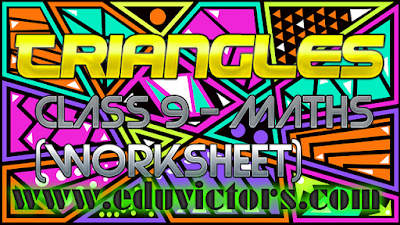## Triangles (Worksheet)

Fill in the blanks

Two figures are _________, if they are of same shape and same size.

If two triangles ABC and XYZ are congruent under the correspondence A ↔ Y, B ↔ X and
C ↔ Z, then symbolically,  △ABC ≊ _________.

If two sides and the included angle of one triangle are equal to two sides and the included angle of the other triangle, then the two triangles are congruent. It is called ________________ rule.

If two angles and the included side of one triangle are equal to two angles and the side of the other triangle, then the two triangles are congruent. It is called ____________ rule.

If two angles and one side of one triangle are equal to two angles and the corresponding side of the other triangle, then the two triangles are congruent. It is called ______________ rule.

If in two right triangles, hypotenuse and one side of a triangle are equal to the hypotenuse and one side of other triangle, then the two triangles are congruent. It is called ______________ rule.

If three sides of one triangle are equal to the three sides of anoter triangle, then the two triangles are congruent. It is called ____________ rule.

Angles opposite to equal sides of a triangle are ________.

Each angle of an equilateral triangle is ______.

Congruent figures have _________ areas.

Of all the line segments that can be drawn to a given line from a point not lying on it, the _________ line segment is the shortest.

In a triangle, side opposite the greater angle is _______.

1. congruent
2. △YXZ
3. SAS Congruence Rule
4. ASA Congruence Rule
5. AAS Congruence Rule
6. RHS congruence Rule
7. SSS Congruence rule
8. Angles opposite to equal sides of a triangle are equal.
9. 60°
10. equal
11. perpendicular
12. longer.